Mathematics Letters
Volume 1, Issue 2, August 2015, Pages: 10-16

On The Prototype Solutions of Symmetric Regularized Long Wave Equation by Generalized Kudryashov Method

Hasan Bulut1, Haci Mehmet Baskonus2, Eren Cüvelek1

1Department of Mathematics, Firat University, Elazig, Turkey

2Department of Computer Engineering, Tunceli University, Tunceli, Turkey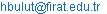(H. Bulut)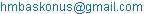(H. M. Baskonus)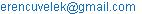(E. Cüvelek)

Hasan Bulut, Haci Mehmet Baskonus, Eren Cüvelek. On The Prototype Solutions of Symmetric Regularized Long Wave Equation By Generalized Kudryashov Method. Mathematics Letters. Vol. 1, No. 2, 2015, pp. 10-16. doi: 10.11648/j.ml.20150102.11

Abstract: In this study, we have applied the generalized kudryashov method to the symmetric regularized long wave equation for obtaining some new analytical solutions such as trigonometric function solution, exponential function solution, complexl function solution, hyperbolic function solution after giving the fundamental properties of method. Afterwards, we have observed that these analytical solutions are verified the symmetric regularized long wave equation by means of Wolfram Mathematica 9. Then, we have drawn two and three dimensional surfaces of analytical solutions. Finally, we have submitted a conclusion to literature.

Keywords: Generalized Kudryashov Method, Symmetric Regularized Long Wave Equation, Exponential Function Solution, Complex Function Solution, Hyperbolic Function Solution, Trigonometric Function Solution

Contents

1. Introduction

All over the world, some researchers have submitted to literature some analytical solutions of many nonlinear partial differential equations. Many powerful methods such as the transformation techniques, the sine–cosine technique, the exponential function method, the standard tanh and developed tanh methods, the jacobi function method, trial equation method, darboux transformation, homotopy perturbation method, sumudu transform method, kudryashov method and so on have been applied successfully [1-11].

When it comes to this paper, we have presented the general properties of generalized kudryashov method (GKM)  in section 2. In section 3, we have applied GKM to the symmetric regularized long wave equation (SRLW) defined by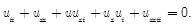(1.1)

Finally, we have submitted a conclusion including some important remarks in section 4.

2. General Properties of Generalized Kudryashov Method

Recently, some authors have constructed the generalized kudryashov method . We consider the following nonlinear partial differential equation for a function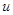of two real variables, space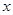and time:(2.1)

The basic phases of the generalized kudryashov method are expressed as the follows:

Step 1. First of all, we must get the travelling wave solution of Eq.(2.1) as following form;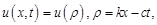(2.2)

whereand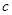are arbitrary constants. Eq.(2.1) was converted into a nonlinear ordinary differential equation of the form:(2.3)

where the prime indicates differentiation with respect to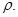Step 2. Suggest that the exact solutions of Eq.(2.3) can be written as the following form;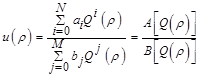(2.4)

where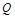is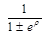. We note that the functionis solution of equation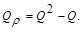(2.5)

Taking into consideration Eq.(2.4), we obtain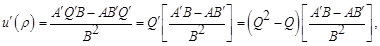(2.6)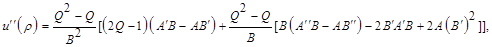(2.7)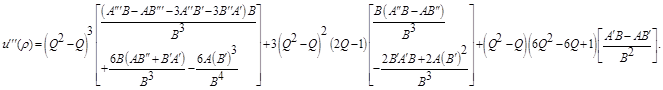(2.8)

Step 3. Under the terms of proposed method, we suppose that the solution of Eq.(2.3) can be explained in the form of the following: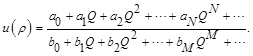(2.9)

To calculate the values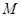and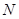in Eq.(2.9) that is the pole order for the general solution of Eq.(2.3), we progress conformably as in the classical kudryashov method on balancing the highest order nonlinear terms in Eq.(2.3) and we  can determine a formula ofand. We can receive some values ofand.

Step 4. Replacing Eq.(2.4) into Eq.(2.3) provides a polynomial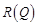of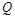. Establishing the coefficients ofto zero, we acquire a system of algebraic equations. Solving this system, we can describe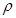and the variable coefficients of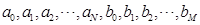. In this way, we attain the exact solutions to Eq.(2.3).

3. Implementation of Method Proposed

For symmetric regularized long wave equation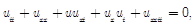(3.1)

with Eq.(2.2) transformation, nonlinear differential form for Eq.(3.1) is obtained as the following;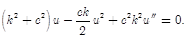(3.2)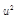and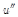terms is used according to balance principle. And then, balance term is obtained as the following;(3.3)

Case 1: For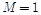and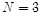when Eq.(2.7) is rewritten with Eq.(2.4), we can find follows;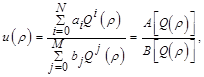(3.4)(3.5)

where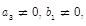.If Eq.(3.4) and Eq.(3.5) are considered in Eq.(3.2), a new equation is occurred with. Solving this equation with Mathematica 9, it yields us the following coefficients;

Case-1.1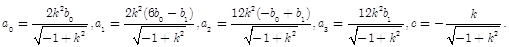(3.6)

If Eq.(3.6) is written in Eq.(3.4), we can obtain the hyperbolic function solution as the following: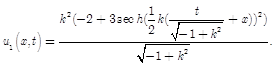(3.7)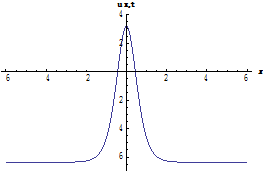Figure 1. Two and three dimensional surfaces of (3.7) hyperbolic function solutions for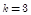and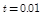for two dimensional surfaces.

Case-1.2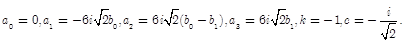(3.8)

If Eq.(3.8) is written in Eq.(3.4), we can obtain being complex trigonometric function solution as the following: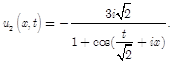(3.9)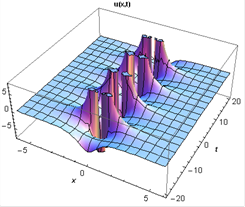Imaginary Part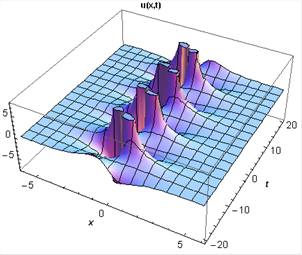Real Part

Figure 2. Three dimensional surfaces of (3.9) the being complex trigonometric function solutions for.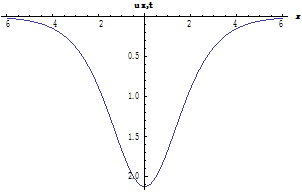Imaginary Part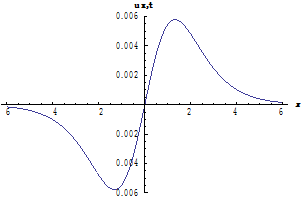Real Part

Figure 3. Two dimensional surfaces of (3.9) being complex trigonometric function solutions for.

Case-1.3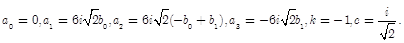(3.10)

If Eq.(3.10) is written in Eq.(3.4), we can obtain being complex trigonometric function solution as the following: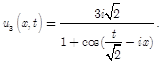(3.11)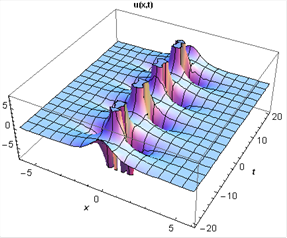Imaginary Part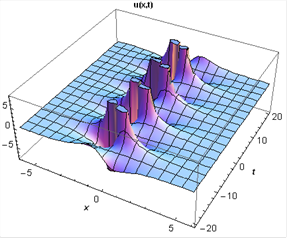Real Part

Figure 4. Three dimensional surfaces of (3.11) being complex trigonometric function solutions for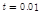.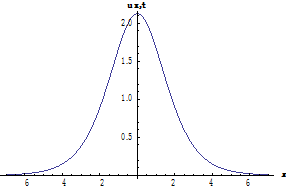Imaginary Part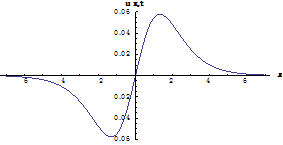Real Part

Figure 5. Two dimensional surfaces of (3.11) being complex trigonometric function solutions for.

Case-1.4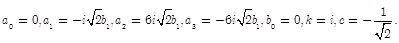(3.12)

If Eq.(3.12) is written in Eq.(3.4), we can obtain complex hyperbolic function solution as the following: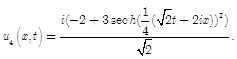(3.13)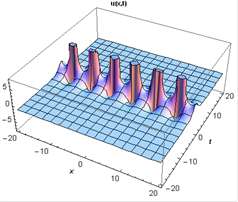Imaginary Part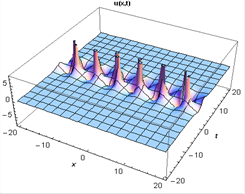Real Part

Figure 6. Three dimensional surfaces of (3.13) complex hyperbolic function solutions for.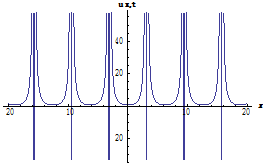Imaginary Part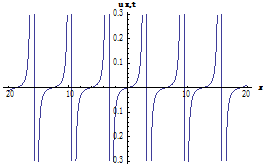Real Part

Figure 7. Two dimensional surfaces of (3.13) complex hyperbolic function solutions for.

Case-1.5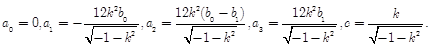(3.14)

If Eq.(3.14) is written in Eq.(3.4), we can obtain complex exponential function solution as the following: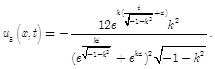(3.15)Imaginary Part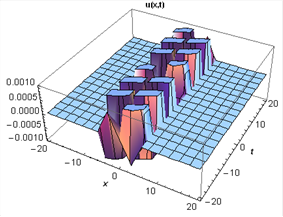Real Part

Figure 8. Three dimensional surfaces of (3.15) complex exponential function solutions for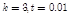.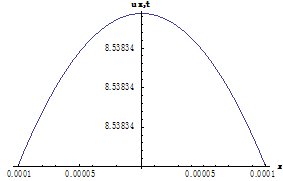Imaginary Part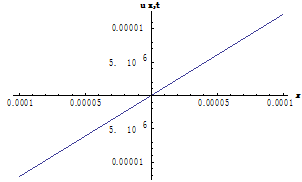Real Part

Figure 9. Two dimensional surfaces of (3.15) complex exponential   function solutions for.

Case-1.6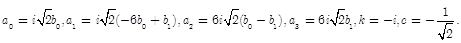(3.16)

If Eq.(3.16) is written in Eq.(3.4), we can obtain complex hyperbolic function solution as the following: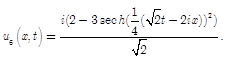(3.17)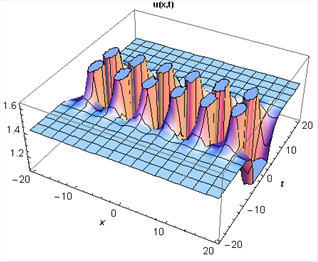Imaginary Part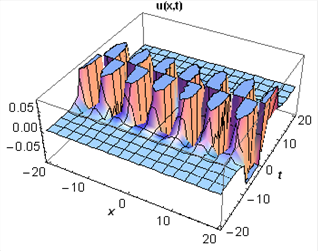Real Part

Figure 10. Three dimensional surfaces of (3.17) complex hyperbolic function solutions for.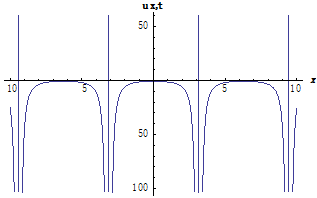Imaginary Part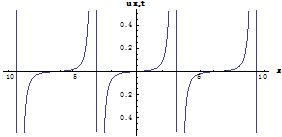Real Part

Figure 11. Two dimensional surfaces of (3.17) complex hyperbolic function solutions for.

4. Conclusions

In this paper, symmetric regularized long wave equation has been solved by using GKM. We have obtained the prototype solutions such as complex function, trigonometric function, exponential function and hyperbolic function solutions. It has been observed that these analytical solutions have verified to the SRLW Eq.(1.1) by using Wolfram Mathematica 9.

These analytical solutions obtained by using GKM in this paper are new complex, trigonometric, exponential and hyperbolic function solutions when we compare these solutions with analytical solutions obtained by Jalil Manafian and Isa Zamanpour . Then, two and three dimensional surfaces of these analytical solutions have been plotted by using Wolfram Mathematica 9.

According to solutions and graphics, one can see that GKM is a powerful tool for obtaining some new analytical solutions for such problems.

Disclosure Statement

No potential conflict of interest was reported by the authors.

References

1. G. K. Watugala, Sumudu Transform: A New Integral Transform to Solve Differantial Equations and Control Engineering Problems, International Journal of Mathematical Education in Science and Technology, 1993, 24, 35-43.
2. Y. Pandir, New exact solutions of the generalized Zakharov–Kuznetsov modified equal-width equation, Pramana journal of physics, 2014, 82(6), 949–964.
3. H. Bulut, H. M. Baskonus and F. B. M. Belgacem, The Analytical Solutions of Some Fractional Ordinary Differential Equations by Sumudu Transform Method, Abstract and Applied Analysis, 2013.
4. A. M. Wazwaz, The tanh method: solitons and periodic solutions for Dodd-Bullough-Mikhailov and Tzitzeica- Dodd-Bullough equations, Chaos, Solitons and Fractals, 2005, 25, 55-56.
5. C.S. Liu, A new trial equation method and its applications, Communications in Theoretical Physics,2006, 45(3), 395-397.
6. C.S. Liu, Trial Equation Method to Nonlinear Evolution Equations with Rank Inhomogeneous: Mathematical Discussions and Its Applications, Communications in Theoretical Physics,2006, 45(2), 219–223.
7. H. Bulut, Y. Pandir, H. M. Baskonus, Symmetrical Hyperbolic Fibonacci Function Solutions of Generalized Fisher Equation with Fractional Order, AIP Conf. Proc.,2013, 1558, 1914 (2013).
8. Y. Pandir, Y. Gurefe, U, Kadak, and E. Misirli, Classifications of exact solutions for some nonlinear partial differential equations with generalized evolution, Abstract and Applied Analysis, vol.2012, Article ID 478531, 16 pages, 2012.
9. Ryabov, P. N., Sinelshchikov, D. I., and Kochanov, M. B., Application of the Kudryashov method for finding exact solutions of the high order nonlinear evolution equations, Applied Mathematics and Computation, 218(7), 3965–3972, (2011).
10. Kudryashov, N. A., One method for finding exact solutions of nonlinear differential equations, Communications in Nonlinear Science and Numerical Simulation, 17(6), 2248–2253, (2012).
11. Lee J., and Sakthivel, R., Exact travelling wave solutions for some important nonlinear physical models, Pramana—Journal of Physics, 80(5), 757–769, (2013).
12. Demiray, S.T., Pandir, Y., and Bulut, H., Generalized Kudryashov Method for Time-Fractional Differential Equations, Abstract and Applied Analysis, 2014, 13 pages, (2014).
13. J. Manafian and I. Zamanpour, Exact travelling wave solutions of the symmetric regularized long wave (SRLW) using analytical methods, Statistics, Optimization And Information Computing, 2, 47–55, 2014.

 Contents 1. 2. 3. 4.
Article ToolsAbstractPDF(1882K)